## Sunday, June 7, 2009

### Polyhedra and Groups

November 21, 2004
I begin with a short review of the properties of regular polyhedra and permutation groups, as well as of the relations between these two areas. These relations led me to the geometric method of multiplying permutations.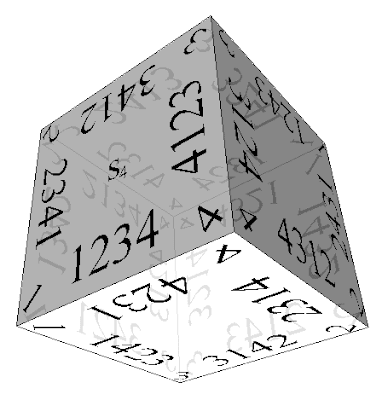Introduction
Regular polyhedra
The regular polyhedra, also known as platonic solids, are represented below: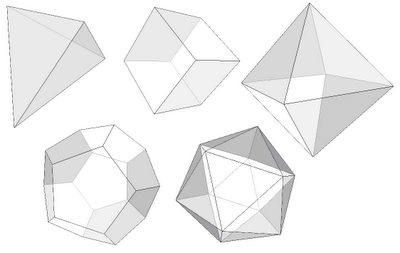Inscribing one regular polyhedron in another

There are many ways to inscribe one regular polyhedron in another. One useful case is that when each vertex of the first polyhedron lies at the center of a face of the second, and each face of the second polyhedron contains one vertex of the first.The cube can be inscribed in this way in the octahedron, but also reciprocally. The same reciprocity holds between the icosahedron and the dodecahedron. Such polyhedra are said to be dual. The tetrahedron is self-dual.
The following ways to inscribe regular polyhedra will be useful too: one or two tetrahedra in a cube, and cube in a dodecahedron.Permutations
You can permute the set {1, 2} in two ways: (1, 2) and (2, 1). What about the set {1, 2, 3}? It is easy to see that we have six possibilities: (1, 2, 3), (2, 3, 1), (3, 1, 2), (2, 1, 3), (3, 2, 1), (1, 3, 2). The result is the same, for any sets of three objects, not only numbers. The number of permutations depends only on the number of elements of the set, and not on their nature. For a set with n elements, this number is 1·2·...·n, and is named the factorial n! of the number n.
An ordered set of the first n positive integers can also be used to express the permutation, as a specific operation to be applied to another ordered set. For example, (2, 1) shows that the elements of an ordered set of two elements are reverted. (2, 3, 1) shows that an ordered set of three, for example (1, 2, 3), changes its order to (2, 3, 1). Such transforms are named permutations of the set {1, 2, ... , n}.

We can multiply two permutations, which means that we apply them successively to the ordered set. All the permutations of a set with n elements forms a grup, because the operation of multiplication of permutations is associative, has a neutral element (which is the identical permutation (1, 2, ... , n), leaving the order unchanged), and every permutation has an inverse which cancels its effect. The group of permutations of the set {1, 2, ... , n} is named the symmetric group of degree n, denoted by $S_n$, and having n! elements.

A transposition is a permutation which interchanges only two elements. For example, (2, 1, 3), (3, 2, 1), (1, 3, 2) are transpositions of the group $S_3$. Any permutation can be decomposed as a product of transpositions in a non unique way. Yet, there is something independent of the way we decompose the permutation as a product of transpositions. If the number of transpositions in such a decomposition is even, it will be even for any other decomposition of the same permutation, and we name such a permutation even. Otherwise, we call it odd. The set of even permutations of a set with n elements forms a subgroup of $S_n$, named the alternating group, denoted by $A_n$, and having n!/2 elements.

The symmetries of a regular polyhedron
By rotating the regular tetrahedron around one of its heights with 120º or 240º, this one remains unchanged. We say that the regular tetrahedron is unchanged by these transformations. We can rotate a regular polyhedron so that, after this transformation, it occupies exactly the same position, but having the faces not necessarily in the same positions. Also, they admit symmetry planes. In fact, this is the idea about the regular polyhedra – their rich symmetry.

The transformations leaving unchanged a polyhedron are named symmetry transformations. One can multiply the transformations. Because each symmetry transformation interchanges the faces of the polyhedron, we can associate to the transformation a permutation from $S_n$, n being the number of faces of the polyhedron. The symmetry transformations leaving invariant a polyhedron form a subgroup of $S_n$, named the automorphism group of the polyhedron.

How many symmetry transformations have each of the regular polyhedra? One easy method to count them is the following. Choose a face a. After a transformation it will take the place of another face a’. Since the polyhedron has n faces, we have n possibilities. Let’s choose a face b, neighbor to the first face before transformation, a. After the transformation, b goes into a face b’, neighbor to the face a’. Each face has the same number of edges k. The face b’ can be one of the k faces neighbor to a’. Therefore, we have nk possibilities. These transforms are the rotations of the polyhedron. But the face a is a polygon, therefore it has two sides. Consequently, when we move the face a in a’, this can flip. In this case, the transformation is no longer a rotation. It can no longer be obtain simply by moving the polyhedron, but by taking its mirror image.

Thus, among all transformations of the polyhedron, a special subgroup is formed by the nk rotations, but the total number of transformations is 2nk. Both these groups are subgroups of $S_n$. For the regular tetrahedron we obtain 2·4·3 = 24 automorphisms, from which the rotations are 4·3 = 12. The cube’s automorphisms group contains 2·6·4 = 48 automorfisms, from which 6·4 = 24 are rotations. The regular octahedron has 2·8·3 = 48 automorfisms, from which 8·3 = 24 rotations, like the cube. Both the icosahedron and the dodecahedron have 2·4·3 = 24 automorfisms, 4·3 = 12 rotations.

We see that the dual polyhedra have the same symmetries.
The symmetries of regular polyhedra and the permutations
Let’s label the vertices of a regular tetrahedron with the numbers {1, 2, 3, 4}: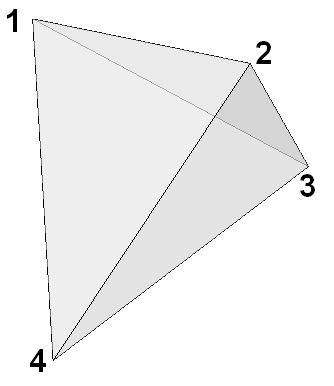At a symmetry transformation, the vertex 1 can go in any of the four vertices. The vertices 2, 3 and 4 are all neighbor with the vertex 1, and they will remain so after the transformation too. Their order around the vertex 1 is preserved in the case of rotations, otherwise it is reverted. Therefore, the vertices’ permutation is even if and only if the transformation is a rotation. The regular tetrahedron having 24 automorphisms, they coincide with the elements of the group $S_4$. The rotations correspond to the elements of the alternating group $A_4$.

Let us now label the cube’s vertices such that the ends of each large diagonal have identical labels from the set {1, 2, 3, 4}, like below:Let’s choose one of the two regular tetrahedra inscribed in the cube. Its vertices are labeled with the numbers {1, 2, 3, 4}. A rotation of the cube rotates also the tetrahedron. Each cube rotation which let the tetrahedron in place corresponds to a rotation of the tetrahedron, and it is an even permutation. The even permutations of the cube’s labels correspond to transformations which interchange the two tetrahedra. Thus, the cube’s rotations correspond to the symmetric group $S_4$.

By labeling the faces of the regular octahedron, we obtain that it has the same symmetry groups as the cube (being its dual).To obtain the symmetry group of the regular dodecahedron, let’s label its edges with the numbers {1, 2, 3, 4, 5}, like in the image: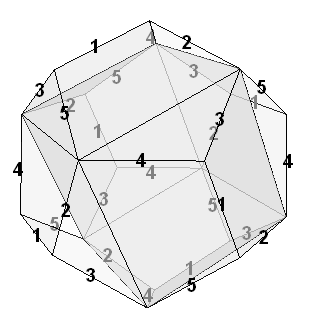For each edge of the dodecahedron, we take the four adjacent edges, and the other vertices of these edges form a square (hint: the four edges are diagonals in identical regular pentagons). These edges form five cubes. Each of the five cubes highlights six of the dodecahedron’s edges. It is easy to see that for each face we obtain a different ordering for the labels. Each rotation of the dodecahedron will take the face labeled by the ordered set (1, 2, 3, 4, 5) (we count starting with the vertex 1) in another face, also labeled with a permutation of the five numbers, so that 1 goes to one number, 2 to another etc. Thus, to the permutation (1, 2, 3, 4, 5) we can associate, as a result of the rotation, another permutation. Because we limit ourselves to rotations, we can choose one orientation (for example clockwise). The rotations will keep this orientation. Because we can start from any vertex of a face when we read the permutation, we will have five permutations for each face. This way, each corner of a face represents a permutation. We can check that these permutations are always even. Each dodecahedron’s rotation corresponds to an even permutation of the face labeled by (1, 2, 3, 4, 5). The dodecahedron’s rotations group is isomorphic with the alternating group $A_5$.
The regular icosahedron being dual to the regular dodecahedron, its 30 edges can be labeled like the ones of the dodecahedron. In this case, we will use cubes with the vertices in the centers of the icosahedron’s faces.

Group operations with polygons
"Calculator" for the Klein group

The Klein’s group has four elements {E, A, B, C}, and its multiplication is given by the label: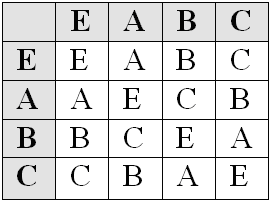This group is isomorphic with the automorphism group of a rectangle. We can construct a „calculator” for multiplying elements of the Klein group by using to identical cards:The first card will be used as witness card. For obtaining all the multiplications with the element B, hence the „multiplication with B table”. For doing this, we rotate the second card (the result card) so that the element B is moved in the position corresponding to the neutral element E of the witness card:Now, to obtain the product of any element X from the group with B, we just read from the result card the element from the position corresponding to the position of X in the witness card. For example, to see the result of the operation C·B, we look for the element C in the witness card. It is in the lower right corner. We look in the same position in the result card. The corresponding element is A. We can check in the multiplicative table that indeed C·B = A.

Calculator for the groups $A_3$ and $S_3$

We start with the group $A_3$ of the even permutations of a set with 3 elements. Its elements are the permutations (1, 2, 3), (2, 3, 1), (3, 1, 2). We construct a card shaped as an equilateral triangle and we label its vertices with the numbers 1, 2 and 3:The rule is: we label the vertices with the numbers {1, 2, 3} clockwise, and the edges with the permutations given by the order in which we met the vertices by starting from that edge and go clockwise.
We see that the rotations with 120º, 240º or 360º preserve this triangle. To find the multiplications of the elements of the $A_3$ with one element of the group, say (3, 1, 2), we keep the witness card in the normal position, and rotate the result card such that the permutation (3, 1, 2) corresponds to the position of the identical permutation (1, 2, 3) from the witness card. In order to find the result of the multiplication of a permutation with the permutation (3, 1, 2), we search on the witness card the position of the desired permutation, and on the result card we just read the permutation on the corresponding position. For example, to find the result of multiplying the permutations (3, 1, 2) and (2, 3, 1), we look on the result card for the permutation corresponding to the position occupied by the permutation (2, 3, 1) on the witness card. We see that the result is the permutation (1, 2, 3):The group $S_3$ contains, in addition to the even permutations (1, 2, 3), (2, 3, 1), (3, 1, 2) from the group $A_3$, the odd permutations (2, 1, 3), (3, 2, 1), (1, 3, 2). This is why we will allow, besides the rotations maintaining the triangle in plane, transformations obtainable by lifting the triangle from the table and flipping it. On its back face we will mark the odd permutations: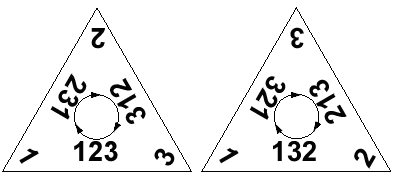Calculator for the groups $C_n$ and $D_n$

A finite group such that all its elements can be obtained by multiplying one element (named generator of the group) with itself, is named cyclic group. We denote the cyclic group with n elements by $C_n$. The cyclic group $C_n$ is isomorphic with the group of integers modulo n, $\mathbb{Z}_n$. The cyclic group C3 is isomorphic with the alternating group $A_3$, but this doesn’t hold for n > 3. We can consider the group $C_n$ as representing the plane rotations of a regular polygon with n edges. If we allow mirror symmetries, obtained by taking the polygon outside the plane and flipping, the number of the possible symmetries doubles, and their group is named the dihedral group, $D_n$. We observe from the definition that for n = 3 the dihedral group is isomorphic to the permutation group of a set of three elements: $D_3$ ~ $S_3$, but, as for the cyclic group, we can’t generalize for n > 3.
The calculators for the cyclic and dihedral groups can be made from regular polyhedra. They can be used similarly to those described for the groups $A_3$ and $S_3$, with a witness polygon and a result polygon.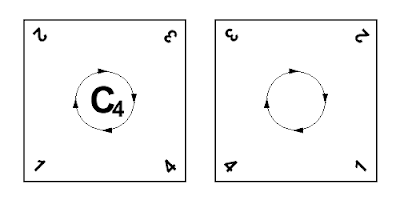Polyhedral calculators of permutations

Calculator for the group $S_4$

Let’s consider again the cube with vertices labeled like this: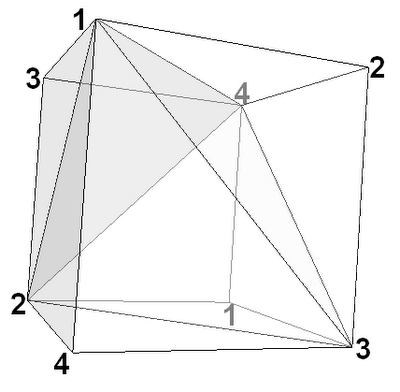Each face has four edges, and we label each of them, on that face, with the permutations obtained by reading the vertices’ labels, starting from that edge and walking clockwise. We obtain a cube labeled on each side of each edge with permutations. The reader can construct her own cube by printing this image:After assembling it in 3D, it will look like this:For finding all the multiplications of the permutations from $S_4$ with a particular permutation, say (4132), we put the witness cube with the face containing the identical permutation (1234) in front, such that the identical permutation is below: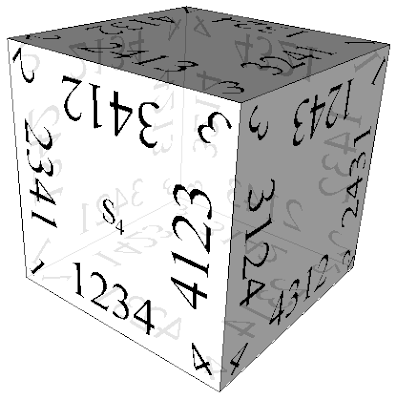Then we put the resulting cube in a similar position, only that on the position of the identical permutation we put the permutation (4132):Let’s suppose we want the result of the multiplication of the permutation (4132) with another permutation from $S_4$, for example (3124). We look in the witness cube the position of the permutation (3124), and in the result cube we read the permutation from the corresponding position. Because the permutation (3124) occurs in the witness cube on the left edge of the right face, we read the permutation from the left edge of the right face of the resulting cube. This is (3412).

Once we position the cubes, we can simply read all the results of the multiplications with the chosen permutation (4132) with any element of $S_4$ on the result cube.

The reader is invited to study the symmetries of the permutations written on the cube.
For example, two permutations associated to the same edge differ by a transposition between the elements labeling the edge’s ends. The permutation (4123) is neighbor of the permutation (3124) – they are on the same edge of the cube. Their common edge has the vertices labeled with the numbers 4 and 3. These numbers are transposed in one of the two permutations to obtain the other. In fact, any two permutations associated to the same edge differ by inverting the positions 1 and 4.

Another interesting property of this cube is that, applying a rotation, we obtain on the initial position of the identical permutation a permutation showing how the four large diagonals of the cube were permuted.

Calculator for the group $A_5$

As we remember, the group of rotations of the dodecahedron is isomorphic with the alternating group $A_5$. We can label the dodecahedron by using even permutations of the set {1, 2, 3, 4, 5}, like this:By printing it and gluing the edges, you can construct a dodecahedron. By constructing two identical such dodecahedra, we can use them to multiply permutations from the alternating group $A_5$, in a similar way we used two cubes to multiply elements of $S_4$.Anonymous said...

hi, very good exposition. can you please tell me where you gathered the material from ? a bibliography ? also, did you actually create the polyhedra yourself out of cartonboard or paper to visualize this ? thanks

Cristi Stoica said...

Thank you.

The way I used polyhedra to represent group multiplication was the result of my own work. The ideas come to me when I studied group representations and the symmetry groups of regular polyhedra. I created the models several years ago on the computer and out of carton.Anonymous said...

hi,im bernard and currently making a thesis paper regarding the permutation of the platonic solids,did the formed cycles from the permutation is disjoint?and how is it related to the ruffini's theorem?

Cristi Stoica said...

Hi Bernard,
this online book.Anonymous said...

Hello, and thank you for the interesting article. The vertices of the dodecahedron can be assigned single digits and the edges assigned the permutation. This is consistent with your cube example and neater. For 6 element permutations the polyhedron becomes instead a plane tiled with hexagons.

cheers,

Doug Barrett
Edmonton

Cristi Stoica said...

Doug,

> The vertices of the dodecahedron can be assigned single digits and the edges assigned the permutation.

I preferred to label the edges to be consistent with Klein's coloring, to use it also in that context.

One can label each vertex of the dodecahedron by starting from the solution presented in the article. One can use for example the following rule: each vertex is labeled with the second digit which appears in all three permutations which meet in that vertex. This rule exploits the fact that all three permutations at the same vertex have the same number on the second position. The resulting group is $A_5$.

> For 6 element permutations the polyhedron becomes instead a plane tiled with hexagons.

Actually, the group $S_6$ is not a subgroup of the group of isometries of the plane. But you can obtain its subgroups of the form $Z_n$ and $D_n$, where $n$ divides $6$.